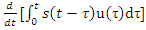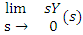# consider a long two wire line composed of soloid round conductors,the radius of both conductors is 0.25 cm and the distance between their centers is 1m.if this distance is doubled then the inductance per unit length

1.  doubles

2.  halves

3.  increases but does not double

4.  decreases but does not halve

4

increases but does not double

Explanation :
No Explanation available for this question

# consider a power system with three identical generators,the transmission losses are negligible.one generator (G1) has a speed governor which maintains its speed constant at the rated value,while the other generators(G2 and G3) have governors with a drop of 5%.if the  load of the system is increased then in steady state.

1.  generation of G2 and G3 is increased equally while generation of G2 and G3 is unchanged

2.  generation of G1 alone is increased while generation of G2 and G3 is unchanged

3.  generation G1,G2 and G3 is increased equally

4.   generation G1,G2 and G3 is increased in the ratio 0.5:0.25:0.25

4

generation of G1 alone is increased while generation of G2 and G3 is unchanged

Explanation :
No Explanation available for this question

# A long wire composed of a smooth round conductor runs above and parallel to the ground(assumed to be a large conducting plane)A high voltage exists between the conductor and the ground,the maximum electric stress occurs at

1.  the upper surface of the conductor

2.  the lower surface of the conductor

3.  the ground surface

4.  midway between the conductor and ground

4

the lower surface of the conductor

Explanation :
No Explanation available for this question

# consider the problem of relay co-ordination for the distance relayas R1 and R2 on adjacent lines of a transmission system.the zone 1 and zone 2 settings for both the relays are indicated on the diagram.which of the following indicates the correct time setting for the zone 2 of relays R1 and R2.

1.  TZ2R1=0.6s,TZ2R2=0.3s

2.  TZ2R1=0.3s,TZ2R2=0.6s

3.  TZ2R1=0.3s,TZ2R2=0.3s

4.  TZ2R1=0.1s,TZ2R2=0.3s

4

TZ2R1=0.6s,TZ2R2=0.3s

Explanation :
No Explanation available for this question

# let s(t) be the step response of a linear system with zero initial conditions then the response of this system to an input u(t) is

1.

2.

3.

4.

4Explanation :
No Explanation available for this question

# let Y(s) be the laplace transformation of the function y(t) then the final value of the function is

1.

2.

3.

4.

4Explanation :
No Explanation available for this question

# the determinant of the matrix

1.  100

2.  200

3.  1

4.  300

4

1

Explanation :
No Explanation available for this question

# the statement transition matrix for the system X=AX with initial state X(0) is

1.  (sI-A)-1

2.  eAT X(0)

3.  Laplace inverse of [(sI-A)-1]

4.  Laplace inverse of [(sI-A)-1Χ(0)]

4

Laplace inverse of [(sI-A)-1]

Explanation :
No Explanation available for this question

# A six pulse thyristor rectifier bridge is connected to a balanced 50Hz three phase ac source.Assuming that the dc output current of the rectifier is constant,the lowest frequency harmonic component in the ac source line current is

1.  100 Hz

2.  150 Hz

3.  250 Hz

4.  300 Hz

4

250 Hz

Explanation :
No Explanation available for this question

1.

2.

3.  200 V

4.  100 V

4